﻿ Math Exercises Generator (High School) - Educational software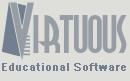ISBN: 978-85-63411-41-9

Language: English## Math Exercises Generator (High School) [Download]

Performs automatic generation of exercises involving various mathematical contents of high school. The software generates thousands of different exercise lists, allowing export them in DOC or PDF format, and personalize with logo and data in the header. You can also choose to display or not the answers. The software generates exercises on the following topics:

 Combinatorics Permutation, arrangement and combination Sets Union, intersection and difference Matrices Addition, subtraction and multiplication Determinants Order 2, 3 and 4 Functions Linear function Quadratic function Polynomial function Inequalities Linear inequalities Quadratic inequalities Exponential equations Modular equations Logarithms Geometry Plane Geometry (areas and perimeters) Solid geometry (areas and volumes) Analytic geometry (lines and circumferences) Linear systems Single unique solution Infinitely many solutions No solution Complex numbers Addition and subtraction Multiplication and division Probability Trigonometry Sine, cosine, tangent Cotangent, secant, cosecant Financial mathematics Simple interest Compound interest Statistics Mode, mean, median, standard deviation and variance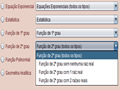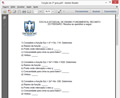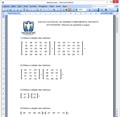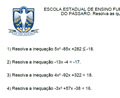# US \$26.00Attention! Download instructions are usually e-mailed within 1 hour to one business day.### Other products

Copyright © 2004-2019 Virtuous Tecnologia Ltda. All rights reserved.